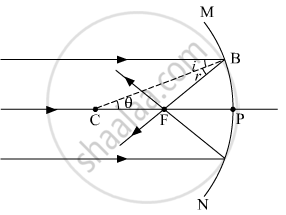# Define the Term 'Focal Length of a Mirror'. with the Help of a Ray Diagram, Obtain the Relation Between Its Focal Length and Radius of Curvature. - Physics

Diagram
Short Note

With the help of a ray diagram, obtain the relation between its focal length and radius of curvature.

#### Solution

The distance between the centre of a lens or curved mirror and its focus.
The relationship between the focal length f and the radius of curvature R = 2f.Consider a ray of light AB, parallel to the principal axis and incident on a spherical mirror at point B. The normal to the surface at point B is CB and CP = CB = R is the radius of curvature. The ray AB, after reflection from a mirror, will pass through F (concave mirror) or will appear to diverge from F (convex mirror) and obeys the law of reflection i.e. i = r.

From the geometry of the figure,
∠BCP = θ = i
In D CBF, θ = r
∴BF = FC (because i = r)
If the aperture of the mirror is small, B lies close to P, and therefore BF = PF
Or FC = FP = PF
Or PC = PF + FC = PF + PF
Or R = 2 PF = 2f
Or f = R/2
Similar relation holds for convex mirror also. In deriving this relation, we have assumed that the aperture of the mirror is small.

Is there an error in this question or solution?
2019-2020 (February) Delhi (Set 2)

Share